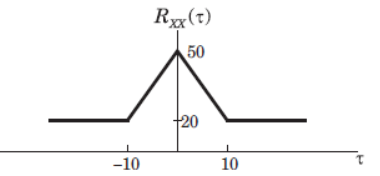Courses

# Test: Random Process

## 10 Questions MCQ Test Electronic Devices | Test: Random Process

Description
This mock test of Test: Random Process for Electrical Engineering (EE) helps you for every Electrical Engineering (EE) entrance exam. This contains 10 Multiple Choice Questions for Electrical Engineering (EE) Test: Random Process (mcq) to study with solutions a complete question bank. The solved questions answers in this Test: Random Process quiz give you a good mix of easy questions and tough questions. Electrical Engineering (EE) students definitely take this Test: Random Process exercise for a better result in the exam. You can find other Test: Random Process extra questions, long questions & short questions for Electrical Engineering (EE) on EduRev as well by searching above.
QUESTION: 1

### For random process X = 6 and Rxx (t, t+t) = 36 + 25 exp(|t|). Consider following statements: (i) X(t) is first order stationary. (ii) X(t) has total average power of 36 W. (iii) X(t) is a wide sense stationary. (iv) X(t) has a periodic component. Q. Which of the following is true?

Solution:

X Constant and Rxx() is not a function of t, so X(t) is a wide sense stationary. So (i) is false & (iii) is true. Pxx = Rxx(0) 36+25 = 61. Thus (ii) is false if X(t) has a periodic component, then RXX(t) will have a periodic component with the same period. Thus (iv) is false.

QUESTION: 2

### White noise with power density No/2 = 6 microW/Hz is applied to an ideal filter of gain 1 and bandwidth W rad/s. If the output’s average noise power is 15 watts, the bandwidth W is

Solution:

Pyy = 1/2p Integral(?xx(w) |H(w)|^2 dw ) from plus infinity to minus infinity. Hence solve for W.

QUESTION: 3

### (Q.3-Q.4) The two-level semi-random binary process is defined by X(t) A or -A where (n 1)T < t < nt and the levels A and -A occur with equal probability. T is a positive constant and n = 0, ±1, ±2. The mean value E[X(t)] is

Solution:

E[X(t)] = A P(A) – (-A)P(-A) which is zero.

QUESTION: 4

The auto correlation Rxx(t1 = 0.5T, t2 = 0.7T) will be

Solution:

Here Rxx is AxA if both t1 and t2 are different and zero if they are same. Hence the answer is AxA.

QUESTION: 5

Air craft of Jet Airways at Ahmedabad airport arrive according to a Poisson process at a rate of 12 per hour. All aircraft are handled by one air traffic controller. If the controller takes a 2 – minute coffee break, what is the probability that he will miss one or more arriving aircraft?

Solution:

P (miss/or more aircraft) = 1 – P(miss 0) = 1 – P(0 arrive).

QUESTION: 6

A stationary random process X(t) is applied to the input of a system for which h(t) = u(t) t2e(-8t). If E[X(t)] = 2, the mean value of the system’s response Y(t) is

Solution:

The mean value of Y(t) is integral of h(t)dt over negative infinity to positive infinity which gives the value equal to 3/128.

QUESTION: 7

A random process is defined by X(t) + A where A is continuous random variable uniformly distributed on(0,1).

The auto correlation function and mean of the process is

Solution:

E[X(t)X(t+t)] = 1/3 and E[X(t)] = 1/2 respectively.

QUESTION: 8

(Q.8-Q.9) The auto correlation function of a stationary ergodic random process is shown below.Q.  The mean value E[X(t)] is

Solution:

Lim |t| tends to infinity, Rxx(t) = 20 = X2. hence X is sqrt(20).

QUESTION: 9

The E[X2(t)] is

Solution:

Rxx(0) = X2 = 50.

QUESTION: 10

The variance is

Solution:

Here X = 0, y = 0, Rxx(0) = 5, Ryy(0) = 10. The only value that satisfies all the given conditions is 30.